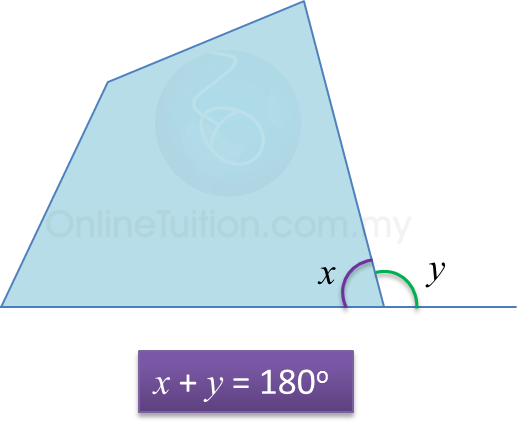# 4.1 Polygons

4.1 Polygons

4.1.1 Regular Polygons
1.  A regular polygon is a polygon where
(a) all its sides are of equal length, and
(b) all its interior angles are of equal size.

2. The number of axis of symmetry of a regular polygon is equal to its number of sides.

Example:4.1.2 Exterior and Interior Angles of Polygons
1. The exterior and interior angles at a vertex of a polygon is supplementary.2. The sum of interior angles of an n-sided polygon is  $\left(n-2\right)×{180}^{o}$

3.
Each interior angle of a regular n-sided polygon is
$\frac{\left(n-2\right)×{180}^{o}}{n}$

4.
The sum of all exterior angles of a polygon is 360o.

5. Each exterior angle of a regular n-sided polygon is
$\frac{{360}^{o}}{n}$